# 405 ECONOMETRICS Chapter 3 TWOVARIABLE REGRESSION MODEL THE

• Slides: 66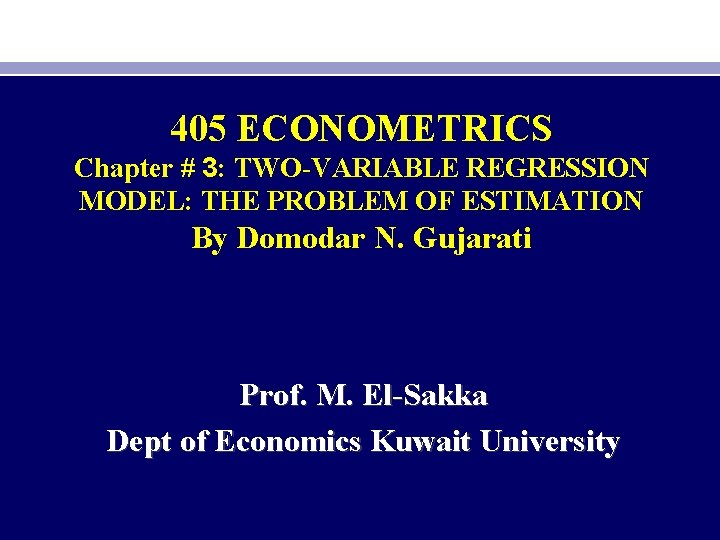405 ECONOMETRICS Chapter # 3: TWO-VARIABLE REGRESSION MODEL: THE PROBLEM OF ESTIMATION By Domodar N. Gujarati Prof. M. El-Sakka Dept of Economics Kuwait University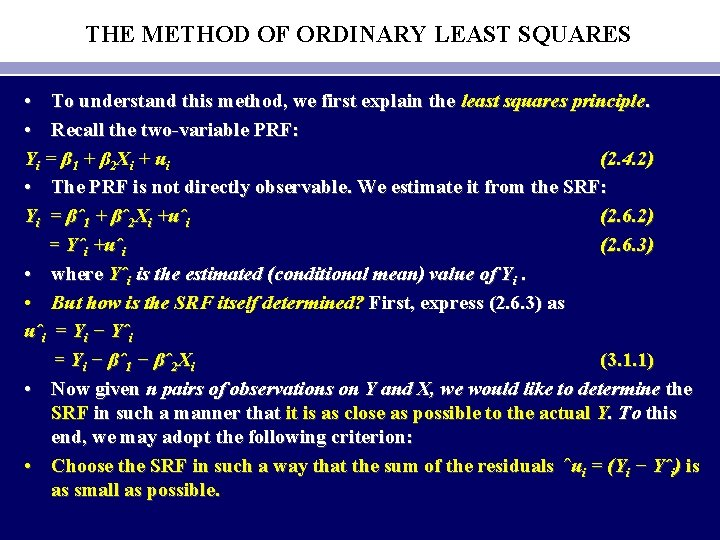THE METHOD OF ORDINARY LEAST SQUARES • To understand this method, we first explain the least squares principle. • Recall the two-variable PRF: Y i = β 1 + β 2 X i + u i (2. 4. 2) • The PRF is not directly observable. We estimate it from the SRF: Yi = βˆ1 + βˆ2 Xi +uˆi (2. 6. 2) = Yˆi +uˆi (2. 6. 3) • where Yˆi is the estimated (conditional mean) value of Yi. • But how is the SRF itself determined? First, express (2. 6. 3) as uˆi = Yi − Yˆi = Yi − βˆ1 − βˆ2 Xi (3. 1. 1) • Now given n pairs of observations on Y and X, we would like to determine the SRF in such a manner that it is as close as possible to the actual Y. To this end, we may adopt the following criterion: • Choose the SRF in such a way that the sum of the residuals ˆui = (Yi − Yˆi) is as small as possible.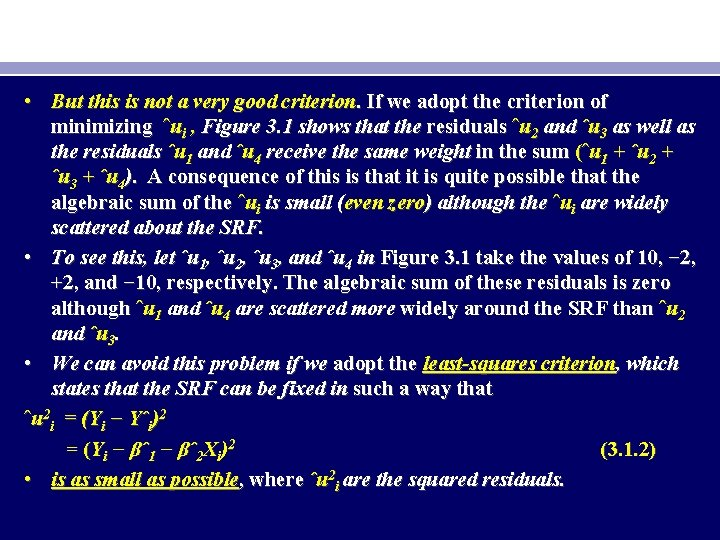• But this is not a very good criterion. If we adopt the criterion of minimizing ˆui , Figure 3. 1 shows that the residuals ˆu 2 and ˆu 3 as well as the residuals ˆu 1 and ˆu 4 receive the same weight in the sum (ˆu 1 + ˆu 2 + ˆu 3 + ˆu 4). A consequence of this is that it is quite possible that the algebraic sum of the ˆui is small (even zero) although the ˆui are widely scattered about the SRF. • To see this, let ˆu 1, ˆu 2, ˆu 3, and ˆu 4 in Figure 3. 1 take the values of 10, − 2, +2, and − 10, respectively. The algebraic sum of these residuals is zero although ˆu 1 and ˆu 4 are scattered more widely around the SRF than ˆu 2 and ˆu 3. • We can avoid this problem if we adopt the least-squares criterion, which states that the SRF can be fixed in such a way that ˆu 2 i = (Yi − Yˆi)2 = (Yi − βˆ1 − βˆ2 Xi)2 (3. 1. 2) • is as small as possible, where ˆu 2 i are the squared residuals.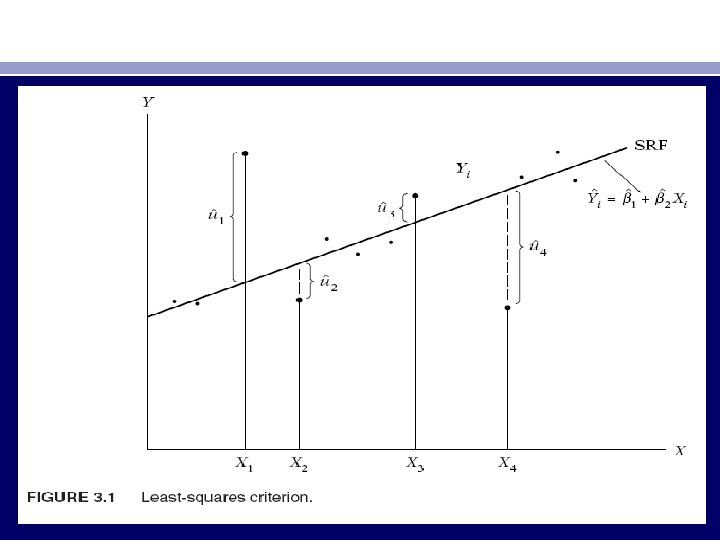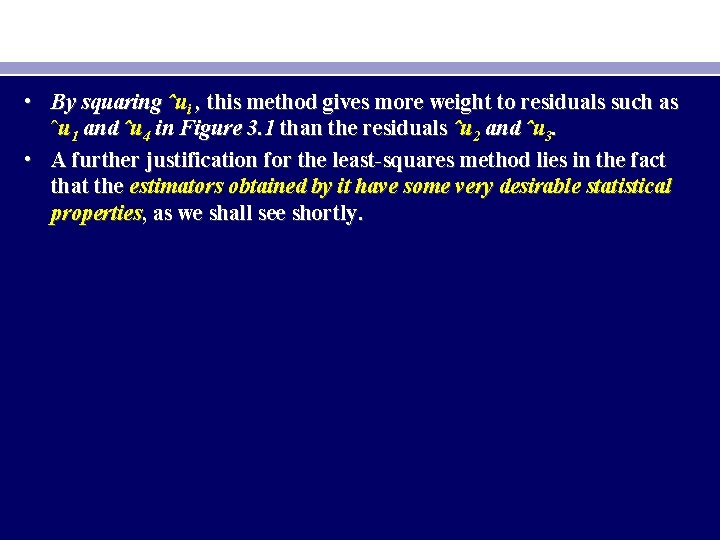• By squaring ˆui , this method gives more weight to residuals such as ˆu 1 and ˆu 4 in Figure 3. 1 than the residuals ˆu 2 and ˆu 3. • A further justification for the least-squares method lies in the fact that the estimators obtained by it have some very desirable statistical properties, as we shall see shortly.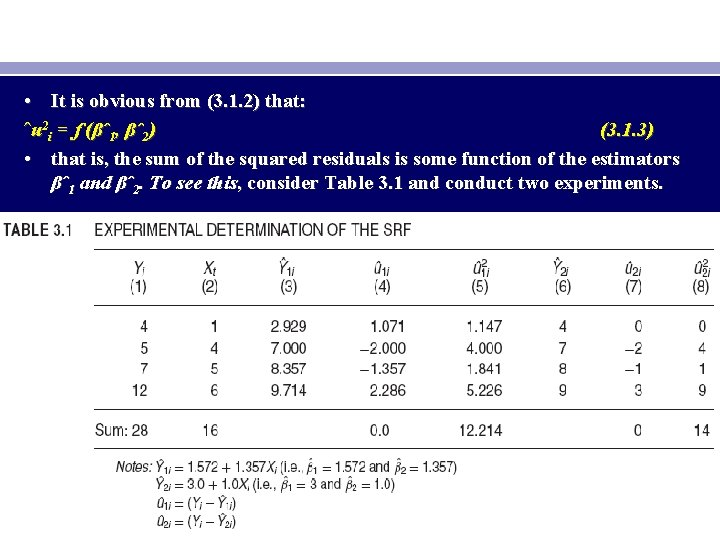• It is obvious from (3. 1. 2) that: ˆu 2 i = f (βˆ1, βˆ2) (3. 1. 3) • that is, the sum of the squared residuals is some function of the estimators βˆ1 and βˆ2. To see this, consider Table 3. 1 and conduct two experiments.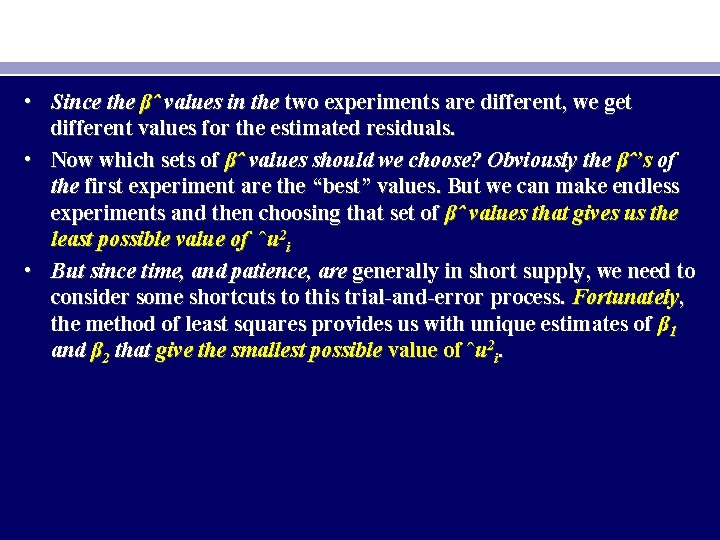• Since the βˆ values in the two experiments are different, we get different values for the estimated residuals. • Now which sets of βˆ values should we choose? Obviously the βˆ’s of the first experiment are the “best” values. But we can make endless experiments and then choosing that set of βˆ values that gives us the least possible value of ˆu 2 i • But since time, and patience, are generally in short supply, we need to consider some shortcuts to this trial-and-error process. Fortunately, the method of least squares provides us with unique estimates of β 1 and β 2 that give the smallest possible value of ˆu 2 i.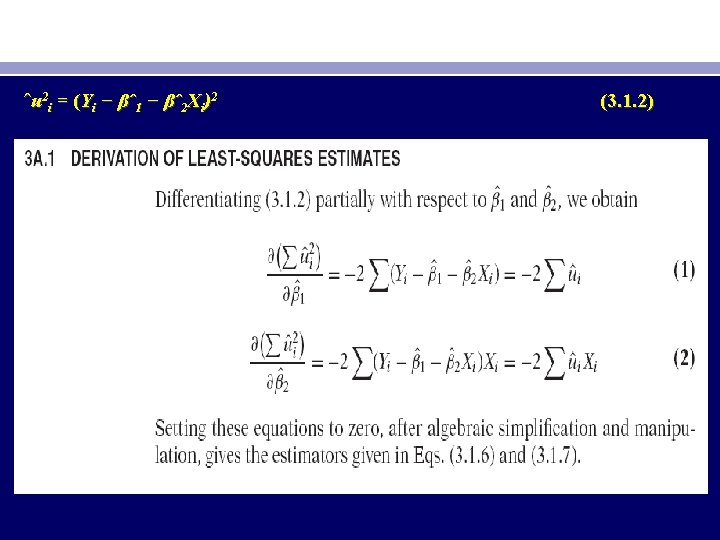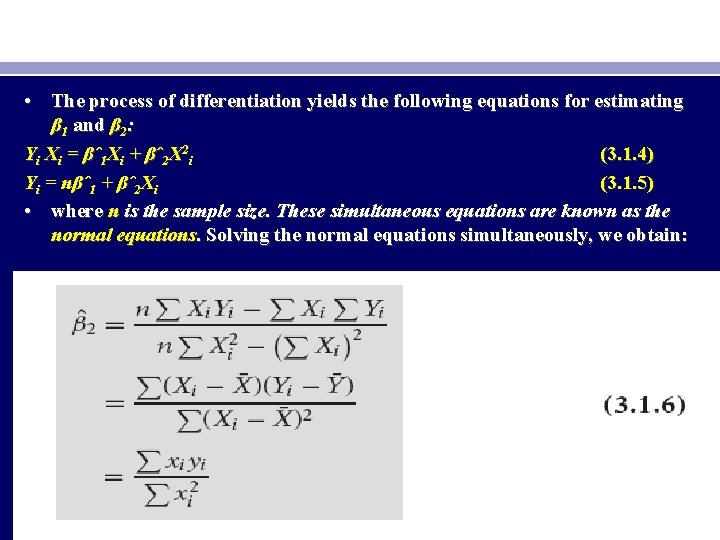• The process of differentiation yields the following equations for estimating β 1 and β 2: Yi Xi = βˆ1 Xi + βˆ2 X 2 i (3. 1. 4) Yi = nβˆ1 + βˆ2 Xi (3. 1. 5) • where n is the sample size. These simultaneous equations are known as the normal equations. Solving the normal equations simultaneously, we obtain: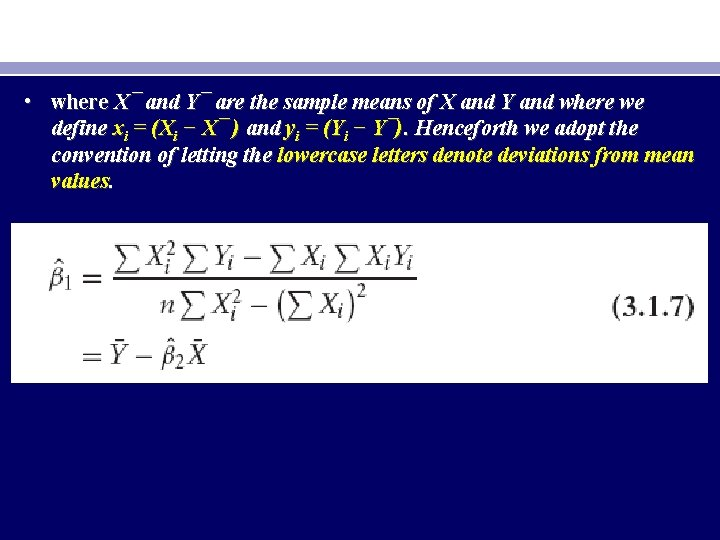• where X¯ and Y¯ are the sample means of X and Y and where we define xi = (Xi − X¯ ) and yi = (Yi − Y¯). Henceforth we adopt the convention of letting the lowercase letters denote deviations from mean values.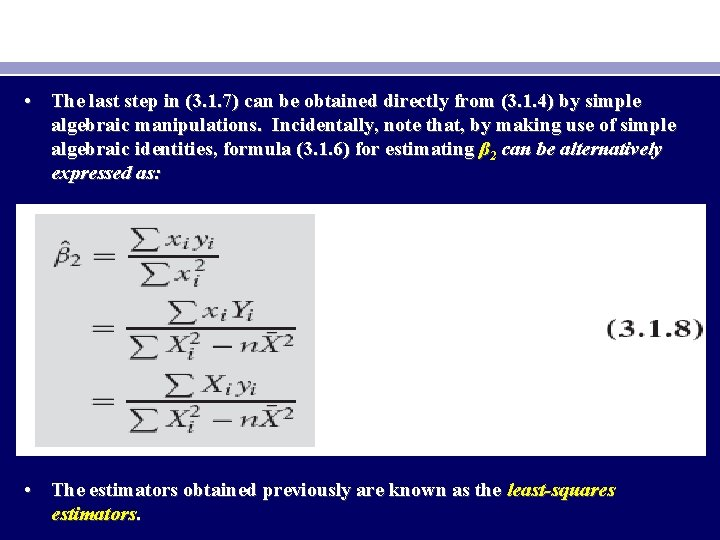• The last step in (3. 1. 7) can be obtained directly from (3. 1. 4) by simple algebraic manipulations. Incidentally, note that, by making use of simple algebraic identities, formula (3. 1. 6) for estimating β 2 can be alternatively expressed as: • The estimators obtained previously are known as the least-squares estimators.• Note the following numerical properties of estimators obtained by the method of OLS: • I. The OLS estimators are expressed solely in terms of the observable (i. e. , sample) quantities (i. e. , X and Y). Therefore, they can be easily computed. • II. They are point estimators; that is, given the sample, each estimator will provide only a single (point, not interval) value of the relevant population parameter. • III. Once the OLS estimates are obtained from the sample data, the sample regression line (Figure 3. 1) can be easily obtained. • The regression line thus obtained has the following properties: – 1. It passes through the sample means of Y and X. This fact is obvious from (3. 1. 7), for the latter can be written as Y¯ = βˆ1 + βˆ2 X¯ , which is shown diagrammatically in Figure 3. 2.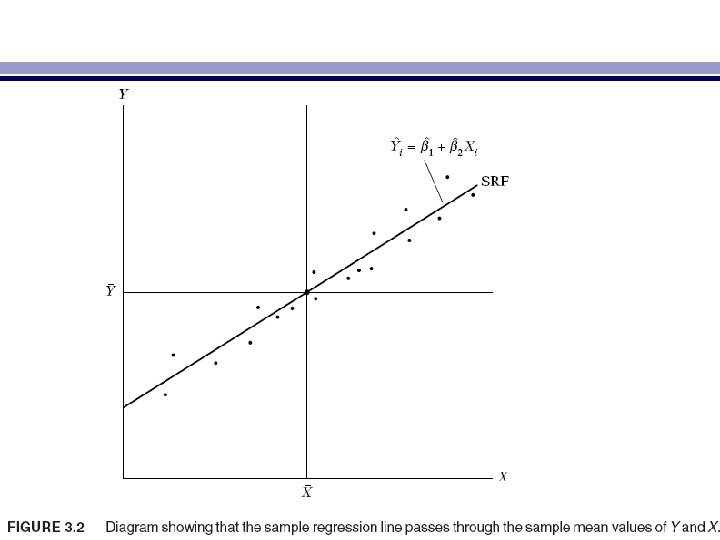– 2. The mean value of the estimated Y = Yˆi is equal to the mean value of the actual Y for: Yˆi = βˆ1 + βˆ2 Xi = (Y¯ − βˆ2 X¯ ) + βˆ2 Xi = Y¯ + βˆ2(Xi − X¯) (3. 1. 9) • Summing both sides of this last equality over the sample values and dividing through by the sample size n gives Y¯ˆ = Y¯ (3. 1. 10) • where use is made of the fact that (Xi − X¯ ) = 0. – 3. The mean value of the residuals ¯ ˆui is zero. From Appendix 3 A, Section 3 A. 1, the first equation is: − 2(Yi − βˆ1 − βˆ2 Xi) = 0 • But since uˆi = Yi − βˆ1 − βˆ2 Xi , the preceding equation reduces to − 2 ˆui = 0, whence ¯ˆu = 0• As a result of the preceding property, the sample regression Yi = βˆ1 + βˆ2 Xi +uˆi (2. 6. 2) • can be expressed in an alternative form where both Y and X are expressed as deviations from their mean values. To see this, sum (2. 6. 2) on both sides to give: Yi = nβˆ1 + βˆ2 Xi +uˆi = nβˆ1 + βˆ2 Xi since uˆi = 0 (3. 1. 11) • Dividing Eq. (3. 1. 11) through by n, we obtain Y¯ = βˆ1 + βˆ2 X¯ (3. 1. 12) • which is the same as (3. 1. 7). Subtracting Eq. (3. 1. 12) from (2. 6. 2), we obtain Yi − Y¯ = βˆ2(Xi − X¯ ) + uˆi • Or yi = βˆ2 xi +uˆi (3. 1. 13)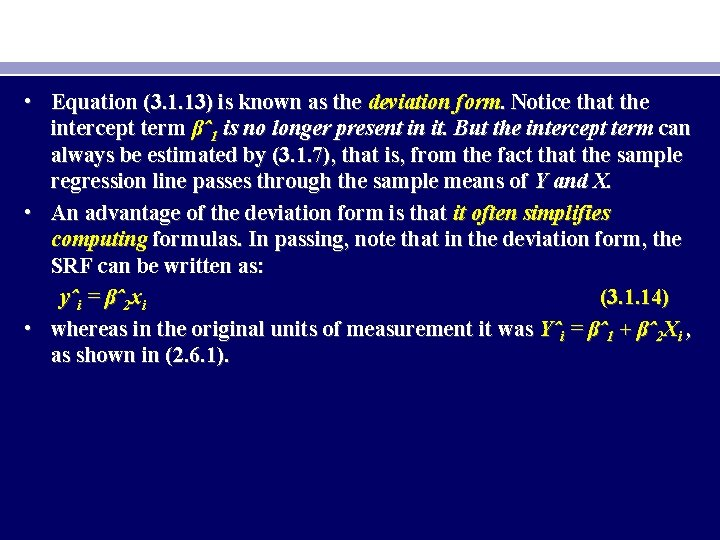• Equation (3. 1. 13) is known as the deviation form. Notice that the intercept term βˆ1 is no longer present in it. But the intercept term can always be estimated by (3. 1. 7), that is, from the fact that the sample regression line passes through the sample means of Y and X. • An advantage of the deviation form is that it often simplifies computing formulas. In passing, note that in the deviation form, the SRF can be written as: yˆi = βˆ2 xi (3. 1. 14) • whereas in the original units of measurement it was Yˆi = βˆ1 + βˆ2 Xi , as shown in (2. 6. 1).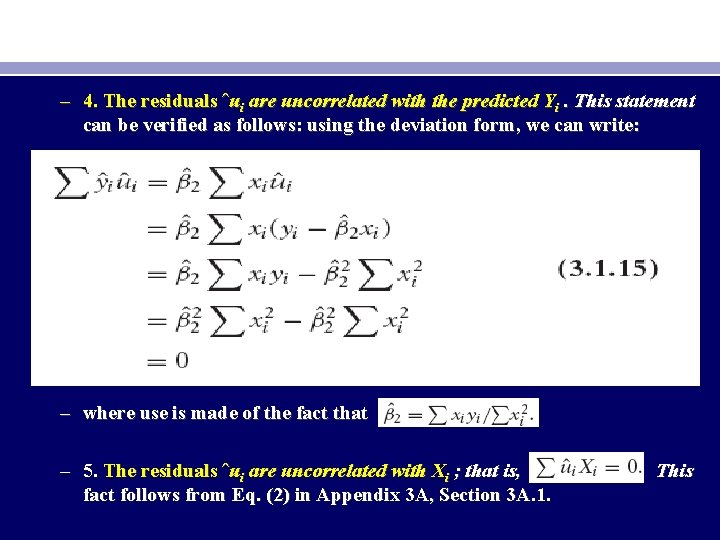– 4. The residuals ˆui are uncorrelated with the predicted Yi. This statement can be verified as follows: using the deviation form, we can write: – where use is made of the fact that – 5. The residuals ˆui are uncorrelated with Xi ; that is, fact follows from Eq. (2) in Appendix 3 A, Section 3 A. 1. This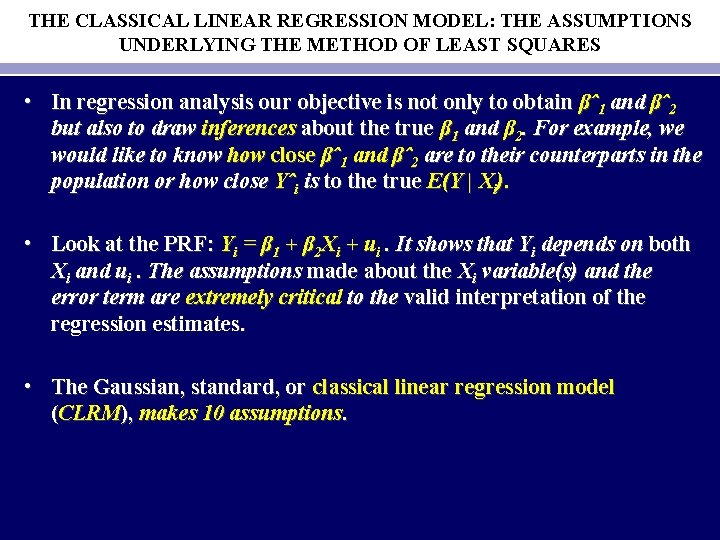THE CLASSICAL LINEAR REGRESSION MODEL: THE ASSUMPTIONS UNDERLYING THE METHOD OF LEAST SQUARES • In regression analysis our objective is not only to obtain βˆ1 and βˆ2 but also to draw inferences about the true β 1 and β 2. For example, we would like to know how close βˆ1 and βˆ2 are to their counterparts in the population or how close Yˆi is to the true E(Y | Xi). • Look at the PRF: Yi = β 1 + β 2 Xi + ui. It shows that Yi depends on both Xi and ui. The assumptions made about the Xi variable(s) and the error term are extremely critical to the valid interpretation of the regression estimates. • The Gaussian, standard, or classical linear regression model (CLRM), makes 10 assumptions.• Keep in mind that the regressand Y and the regressor X themselves may be nonlinear.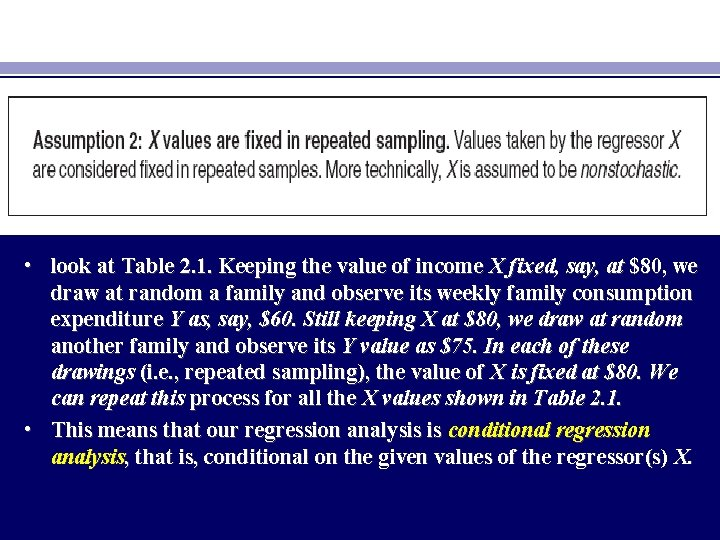• look at Table 2. 1. Keeping the value of income X fixed, say, at \$80, we draw at random a family and observe its weekly family consumption expenditure Y as, say, \$60. Still keeping X at \$80, we draw at random another family and observe its Y value as \$75. In each of these drawings (i. e. , repeated sampling), the value of X is fixed at \$80. We can repeat this process for all the X values shown in Table 2. 1. • This means that our regression analysis is conditional regression analysis, that is, conditional on the given values of the regressor(s) X.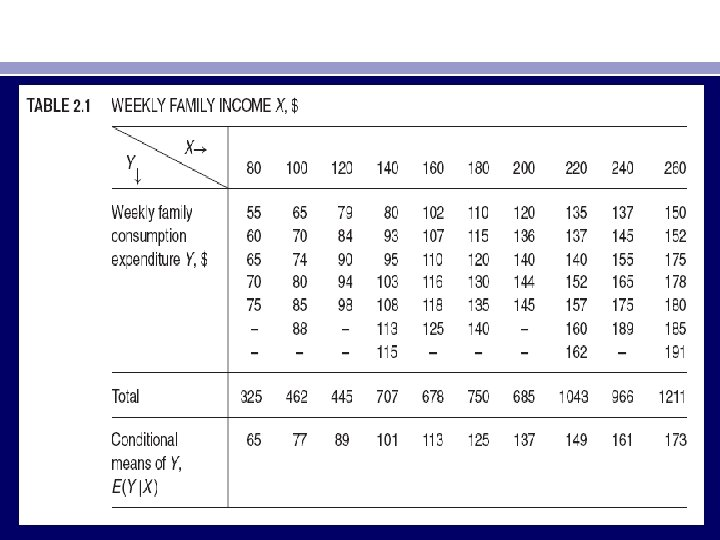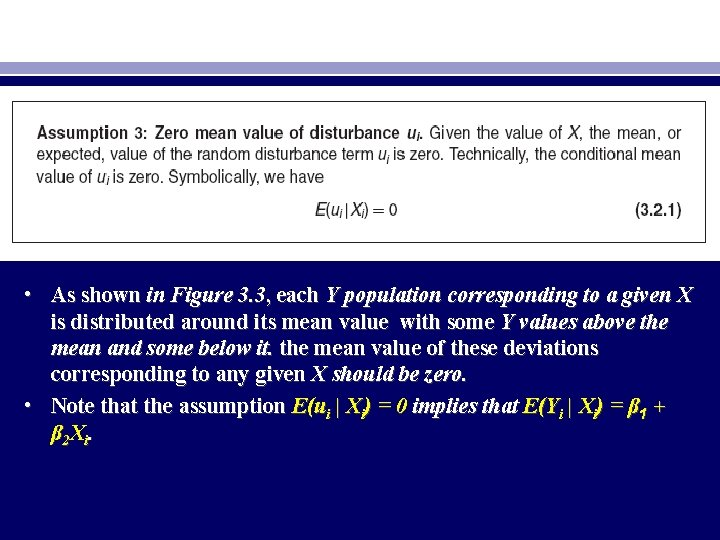• As shown in Figure 3. 3, each Y population corresponding to a given X is distributed around its mean value with some Y values above the mean and some below it. the mean value of these deviations corresponding to any given X should be zero. • Note that the assumption E(ui | Xi) = 0 implies that E(Yi | Xi) = β 1 + β 2 Xi.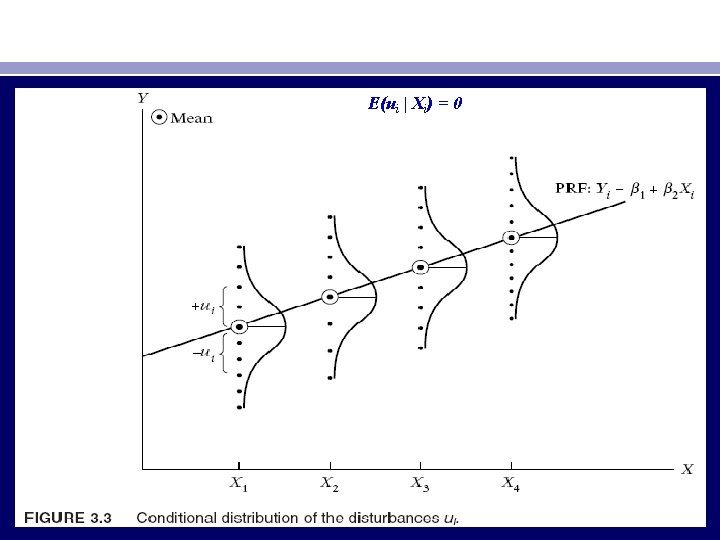E(ui | Xi) = 0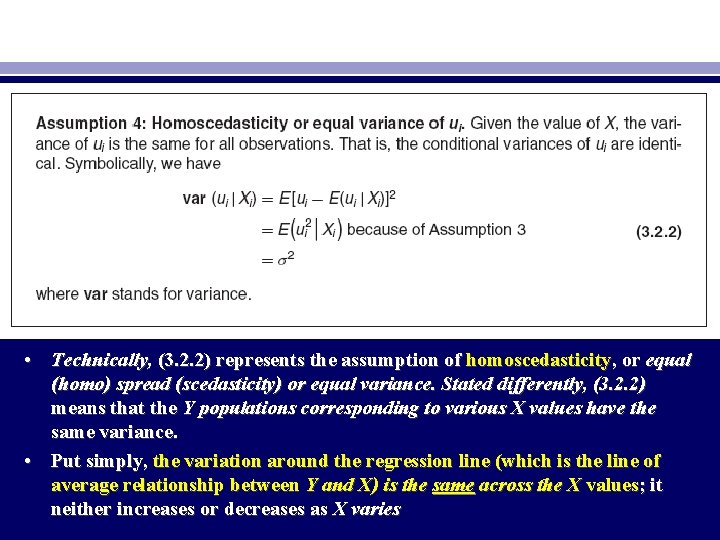• Technically, (3. 2. 2) represents the assumption of homoscedasticity, or equal (homo) spread (scedasticity) or equal variance. Stated differently, (3. 2. 2) means that the Y populations corresponding to various X values have the same variance. • Put simply, the variation around the regression line (which is the line of average relationship between Y and X) is the same across the X values; it neither increases or decreases as X varies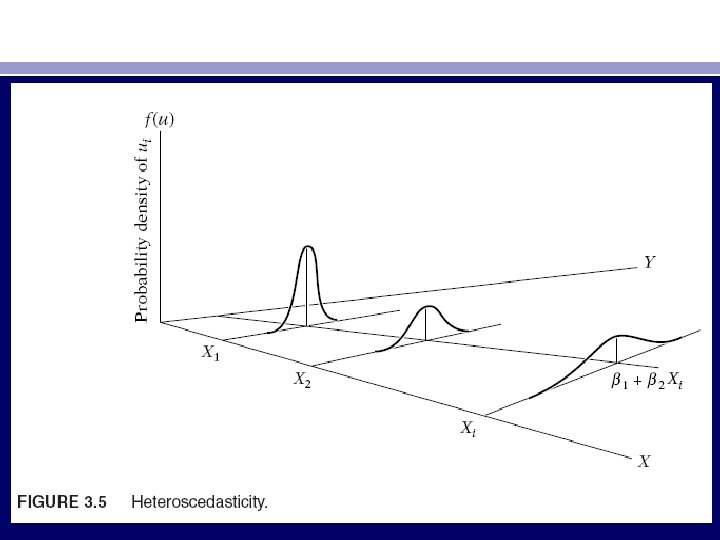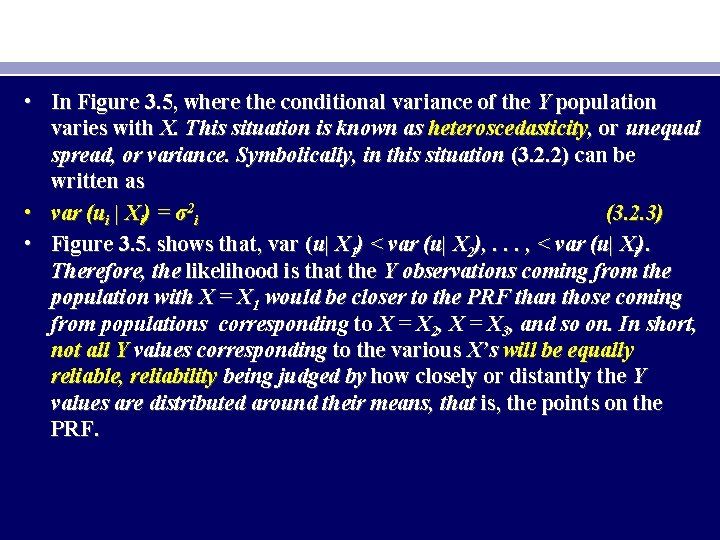• In Figure 3. 5, where the conditional variance of the Y population varies with X. This situation is known as heteroscedasticity, or unequal spread, or variance. Symbolically, in this situation (3. 2. 2) can be written as • var (ui | Xi) = σ2 i (3. 2. 3) • Figure 3. 5. shows that, var (u| X 1) < var (u| X 2), . . . , < var (u| Xi). Therefore, the likelihood is that the Y observations coming from the population with X = X 1 would be closer to the PRF than those coming from populations corresponding to X = X 2, X = X 3, and so on. In short, not all Y values corresponding to the various X’s will be equally reliable, reliability being judged by how closely or distantly the Y values are distributed around their means, that is, the points on the PRF.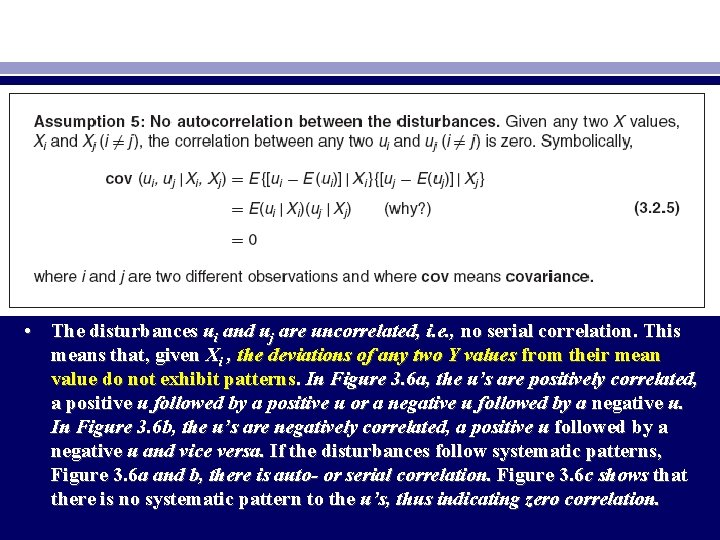• The disturbances ui and uj are uncorrelated, i. e. , no serial correlation. This means that, given Xi , the deviations of any two Y values from their mean value do not exhibit patterns. In Figure 3. 6 a, the u’s are positively correlated, a positive u followed by a positive u or a negative u followed by a negative u. In Figure 3. 6 b, the u’s are negatively correlated, a positive u followed by a negative u and vice versa. If the disturbances follow systematic patterns, Figure 3. 6 a and b, there is auto- or serial correlation. Figure 3. 6 c shows that there is no systematic pattern to the u’s, thus indicating zero correlation.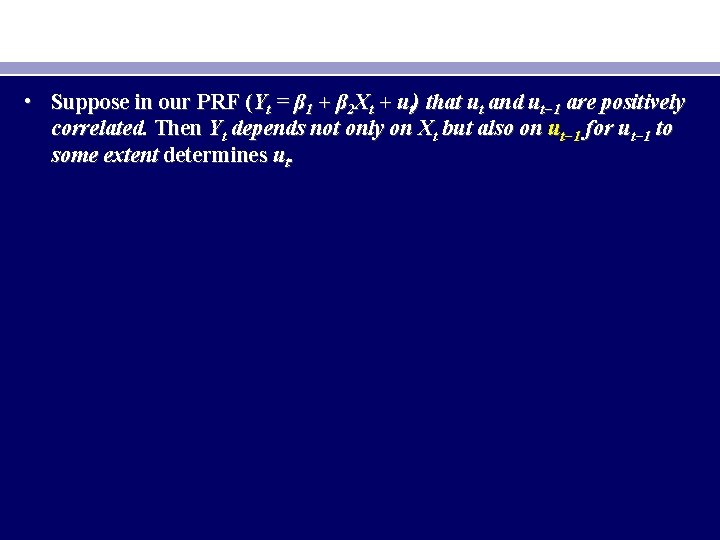• Suppose in our PRF (Yt = β 1 + β 2 Xt + ut) that ut and ut− 1 are positively correlated. Then Yt depends not only on Xt but also on ut− 1 for ut− 1 to some extent determines ut.• The disturbance u and explanatory variable X are uncorrelated. The PRF assumes that X and u (which may represent the influence of all the omitted variables) have separate (and additive) influence on Y. But if X and u are correlated, it is not possible to assess their individual effects on Y. Thus, if X and u are positively correlated, X increases when u increases and it decreases when u decreases. Similarly, if X and u are negatively correlated, X increases when u decreases and it decreases when u increases. In either case, it is difficult to isolate the influence of X and u on Y.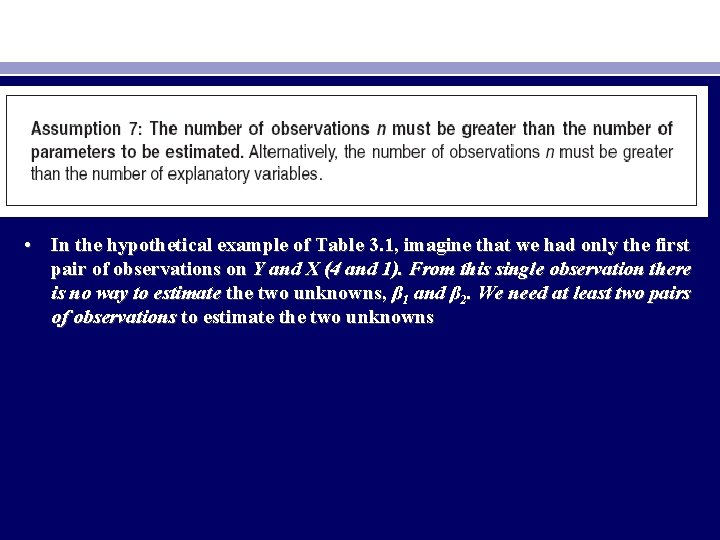• In the hypothetical example of Table 3. 1, imagine that we had only the first pair of observations on Y and X (4 and 1). From this single observation there is no way to estimate the two unknowns, β 1 and β 2. We need at least two pairs of observations to estimate the two unknowns• This assumption too is not so innocuous as it looks. Look at Eq. (3. 1. 6). If all the X values are identical, then Xi = X¯ and the denominator of that equation will be zero, making it impossible to estimate β 2 and therefore β 1. Looking at our family consumption expenditure example in Chapter 2, if there is very little variation in family income, we will not be able to explain much of the variation in the consumption expenditure.• An econometric investigation begins with the specification of the econometric model underlying the phenomenon of interest. Some important questions that arise in the specification of the model include the following: (1) What variables should be included in the model? • (2) What is the functional form of the model? Is it linear in the parameters, the variables, or both? • (3) What are the probabilistic assumptions made about the Yi , the Xi, and the ui entering the model?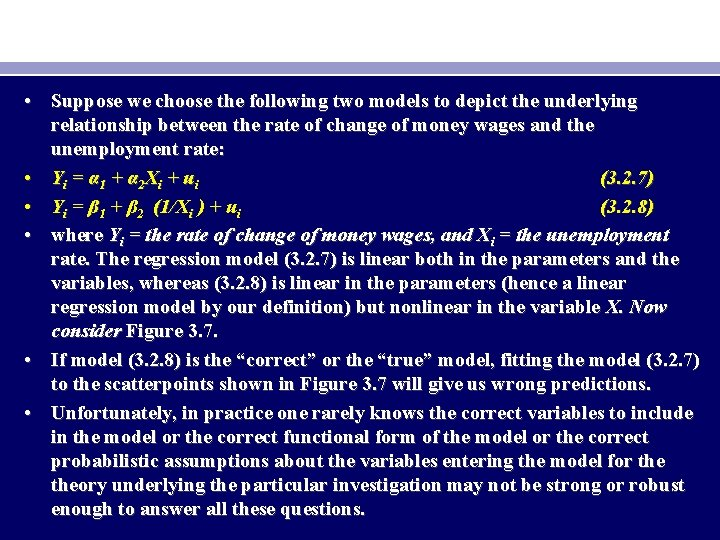• Suppose we choose the following two models to depict the underlying relationship between the rate of change of money wages and the unemployment rate: • Y i = α 1 + α 2 X i + u i (3. 2. 7) • Yi = β 1 + β 2 (1/Xi ) + ui (3. 2. 8) • where Yi = the rate of change of money wages, and Xi = the unemployment rate. The regression model (3. 2. 7) is linear both in the parameters and the variables, whereas (3. 2. 8) is linear in the parameters (hence a linear regression model by our definition) but nonlinear in the variable X. Now consider Figure 3. 7. • If model (3. 2. 8) is the “correct” or the “true” model, fitting the model (3. 2. 7) to the scatterpoints shown in Figure 3. 7 will give us wrong predictions. • Unfortunately, in practice one rarely knows the correct variables to include in the model or the correct functional form of the model or the correct probabilistic assumptions about the variables entering the model for theory underlying the particular investigation may not be strong or robust enough to answer all these questions.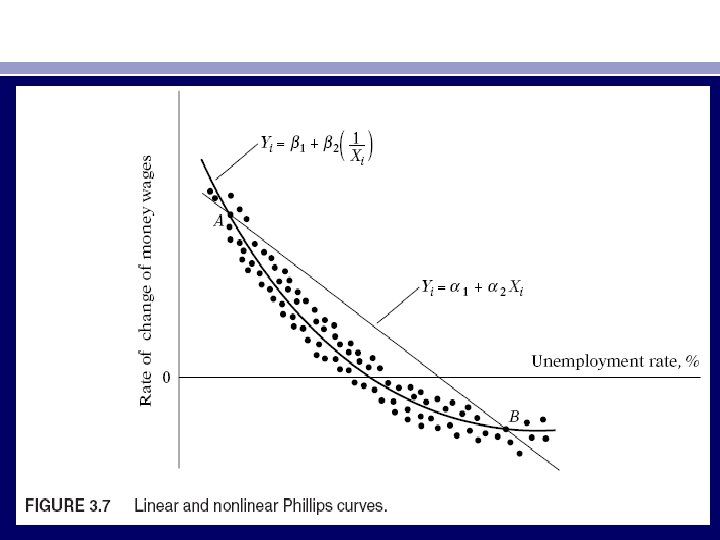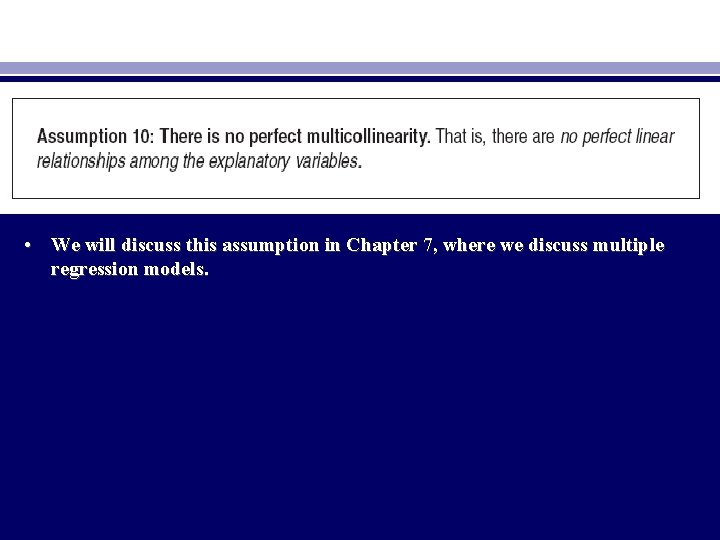• We will discuss this assumption in Chapter 7, where we discuss multiple regression models.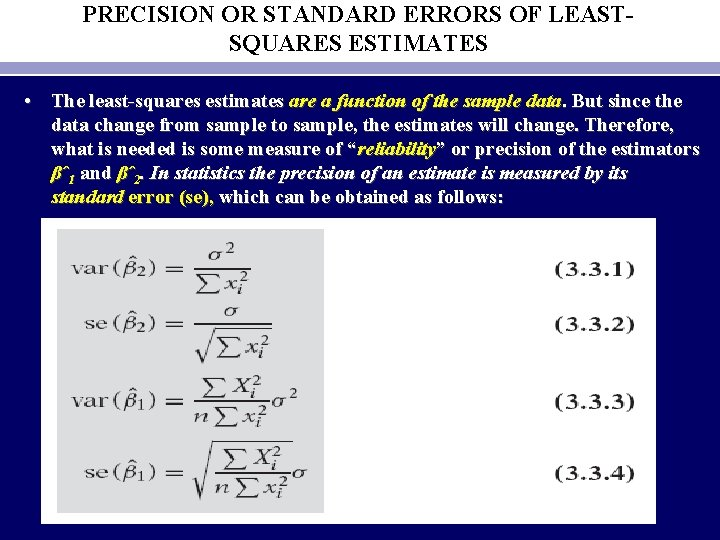PRECISION OR STANDARD ERRORS OF LEASTSQUARES ESTIMATES • The least-squares estimates are a function of the sample data. But since the data change from sample to sample, the estimates will change. Therefore, what is needed is some measure of “reliability” or precision of the estimators βˆ1 and βˆ2. In statistics the precision of an estimate is measured by its standard error (se), which can be obtained as follows:• σ2 is the constant or homoscedastic variance of ui of Assumption 4. • σ2 itself is estimated by the following formula: • where ˆσ2 is the OLS estimator of the true but unknown σ2 and where the expression n− 2 is known as the number of degrees of freedom (df), is the residual sum of squares (RSS). Once is known, ˆσ2 can be easily computed. • Compared with Eq. (3. 1. 2), Eq. (3. 3. 6) is easy to use, for it does not require computing ˆui for each observation.• Since • an alternative expression for computing is • In passing, note that the positive square root of ˆσ2 • is known as the standard error of estimate or the standard error of the regression (se). It is simply the standard deviation of the Y values about the estimated regression line and is often used as a summary measure of the “goodness of fit” of the estimated regression line.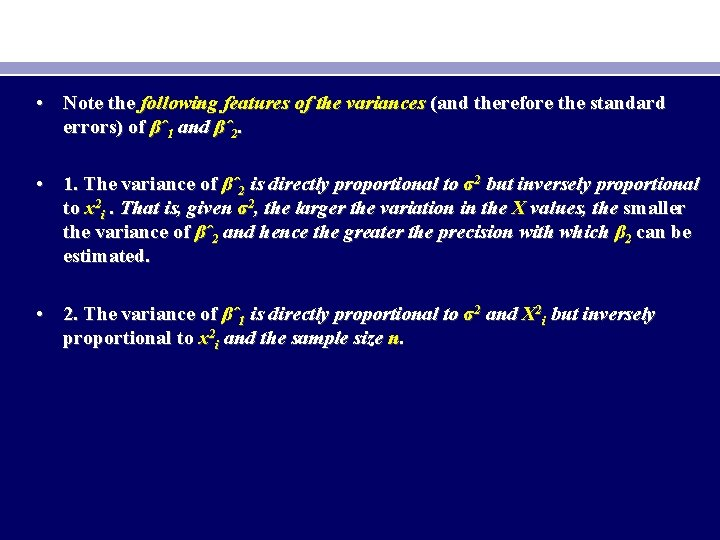• Note the following features of the variances (and therefore the standard errors) of βˆ1 and βˆ2. • 1. The variance of βˆ2 is directly proportional to σ2 but inversely proportional to x 2 i. That is, given σ2, the larger the variation in the X values, the smaller the variance of βˆ2 and hence the greater the precision with which β 2 can be estimated. • 2. The variance of βˆ1 is directly proportional to σ2 and X 2 i but inversely proportional to x 2 i and the sample size n.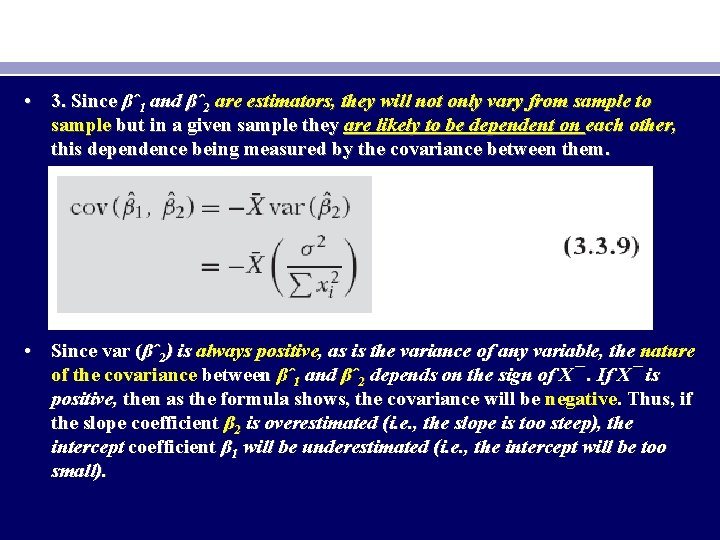• 3. Since βˆ1 and βˆ2 are estimators, they will not only vary from sample to sample but in a given sample they are likely to be dependent on each other, this dependence being measured by the covariance between them. • Since var (βˆ2) is always positive, as is the variance of any variable, the nature of the covariance between βˆ1 and βˆ2 depends on the sign of X¯. If X¯ is positive, then as the formula shows, the covariance will be negative. Thus, if the slope coefficient β 2 is overestimated (i. e. , the slope is too steep), the intercept coefficient β 1 will be underestimated (i. e. , the intercept will be too small).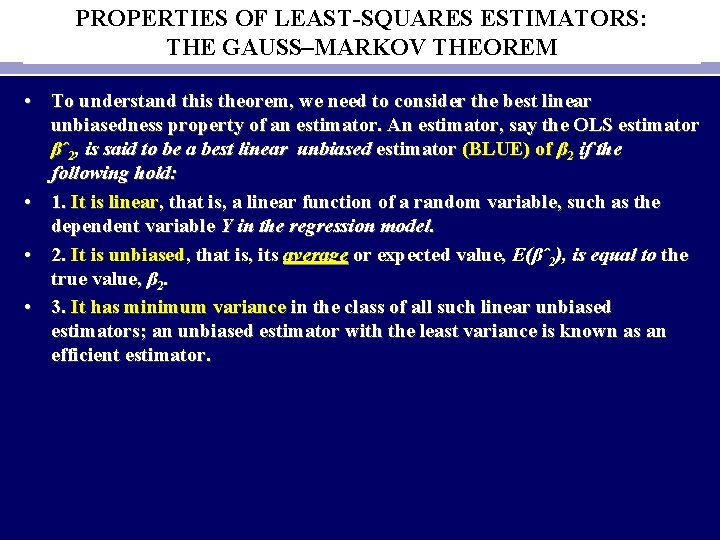PROPERTIES OF LEAST-SQUARES ESTIMATORS: THE GAUSS–MARKOV THEOREM • To understand this theorem, we need to consider the best linear unbiasedness property of an estimator. An estimator, say the OLS estimator βˆ2, is said to be a best linear unbiased estimator (BLUE) of β 2 if the following hold: • 1. It is linear, that is, a linear function of a random variable, such as the dependent variable Y in the regression model. • 2. It is unbiased, that is, its average or expected value, E(βˆ2), is equal to the true value, β 2. • 3. It has minimum variance in the class of all such linear unbiased estimators; an unbiased estimator with the least variance is known as an efficient estimator.• What all this means can be explained with the aid of Figure 3. 8. In Figure 3. 8(a) we have shown the sampling distribution of the OLS estimator βˆ2, that is, the distribution of the values taken by βˆ2 in repeated sampling experiment. For convenience we have assumed βˆ2 to be distributed symmetrically. As the figure shows, the mean of the βˆ2 values, E(βˆ2), is equal to the true β 2. In this situation we say that βˆ2 is an unbiased estimator of β 2. In Figure 3. 8(b) we have shown the sampling distribution of β∗ 2, an alternative estimator of β 2 obtained by using another (i. e. , other than OLS) method.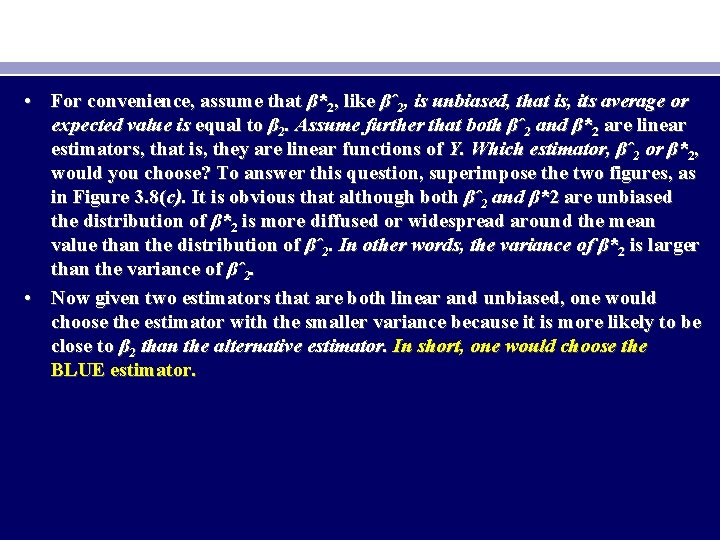• For convenience, assume that β*2, like βˆ2, is unbiased, that is, its average or expected value is equal to β 2. Assume further that both βˆ2 and β*2 are linear estimators, that is, they are linear functions of Y. Which estimator, βˆ2 or β*2, would you choose? To answer this question, superimpose the two figures, as in Figure 3. 8(c). It is obvious that although both βˆ2 and β*2 are unbiased the distribution of β*2 is more diffused or widespread around the mean value than the distribution of βˆ2. In other words, the variance of β*2 is larger than the variance of βˆ2. • Now given two estimators that are both linear and unbiased, one would choose the estimator with the smaller variance because it is more likely to be close to β 2 than the alternative estimator. In short, one would choose the BLUE estimator.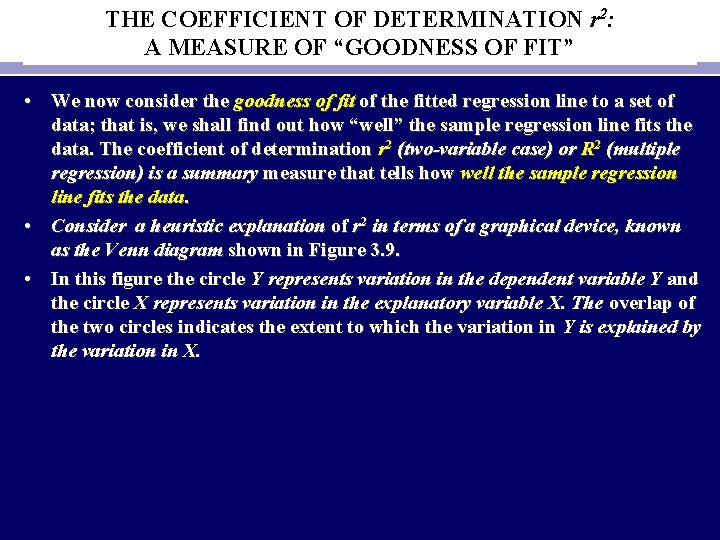THE COEFFICIENT OF DETERMINATION r 2: A MEASURE OF “GOODNESS OF FIT” • We now consider the goodness of fit of the fitted regression line to a set of data; that is, we shall find out how “well” the sample regression line fits the data. The coefficient of determination r 2 (two-variable case) or R 2 (multiple regression) is a summary measure that tells how well the sample regression line fits the data. • Consider a heuristic explanation of r 2 in terms of a graphical device, known as the Venn diagram shown in Figure 3. 9. • In this figure the circle Y represents variation in the dependent variable Y and the circle X represents variation in the explanatory variable X. The overlap of the two circles indicates the extent to which the variation in Y is explained by the variation in X.• • • To compute this r 2, we proceed as follows: Recall that Yi = Yˆi +uˆi (2. 6. 3) or in the deviation form yi = ˆyi + ˆui (3. 5. 1) where use is made of (3. 1. 13) and (3. 1. 14). Squaring (3. 5. 1) on both sides and summing over the sample, we obtain • • Since = 0 and yˆi = βˆ2 xi.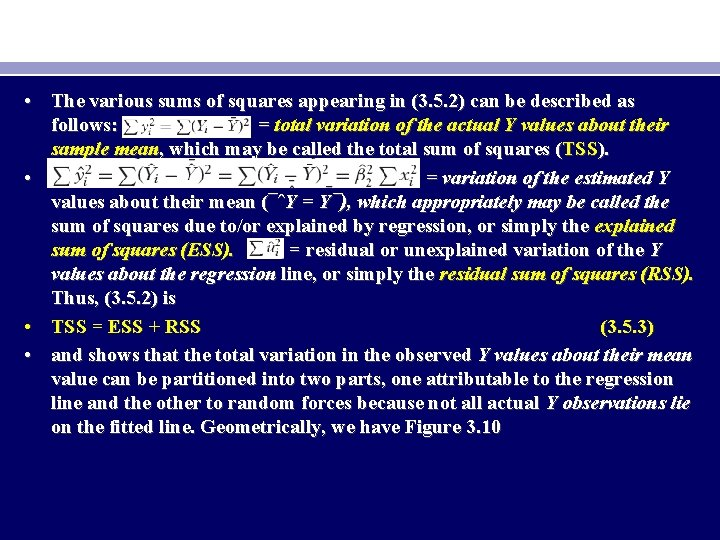• The various sums of squares appearing in (3. 5. 2) can be described as follows: = total variation of the actual Y values about their sample mean, which may be called the total sum of squares (TSS). • = variation of the estimated Y values about their mean (¯ˆY = Y¯), which appropriately may be called the sum of squares due to/or explained by regression, or simply the explained sum of squares (ESS). = residual or unexplained variation of the Y values about the regression line, or simply the residual sum of squares (RSS). Thus, (3. 5. 2) is • TSS = ESS + RSS (3. 5. 3) • and shows that the total variation in the observed Y values about their mean value can be partitioned into two parts, one attributable to the regression line and the other to random forces because not all actual Y observations lie on the fitted line. Geometrically, we have Figure 3. 10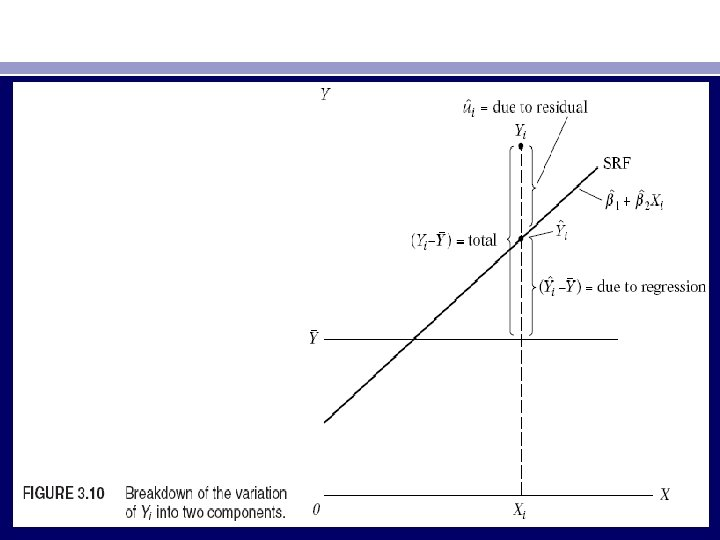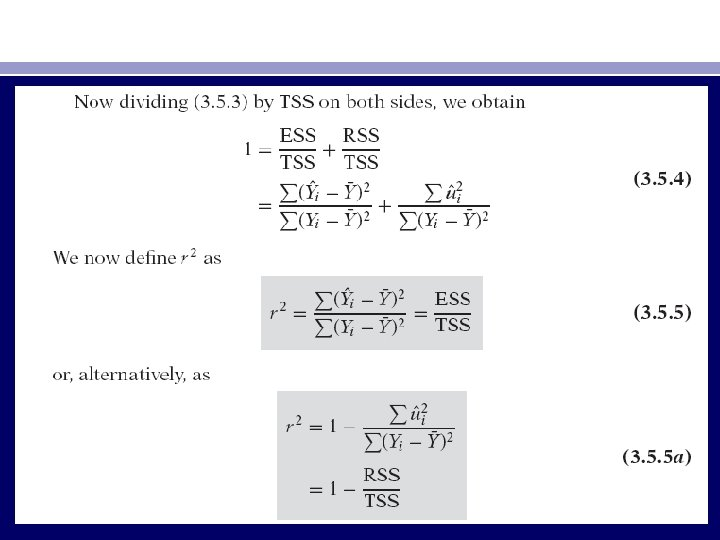• The quantity r 2 thus defined is known as the (sample) coefficient of determination and is the most commonly used measure of the goodness of fit of a regression line. Verbally, r 2 measures the proportion or percentage of the total variation in Y explained by the regression model. • Two properties of r 2 may be noted: • 1. It is a nonnegative quantity. • 2. Its limits are 0 ≤ r 2 ≤ 1. An r 2 of 1 means a perfect fit, that is, Yˆi = Yi for each i. On the other hand, an r 2 of zero means that there is no relationship between the regressand the regressor whatsoever (i. e. , βˆ2 = 0). In this case, as (3. 1. 9) shows, Yˆi = βˆ1 = Y¯, that is, the best prediction of any Y value is simply its mean value. In this situation therefore the regression line will be horizontal to the X axis. • Although r 2 can be computed directly from its definition given in (3. 5. 5), it can be obtained more quickly from the following formula: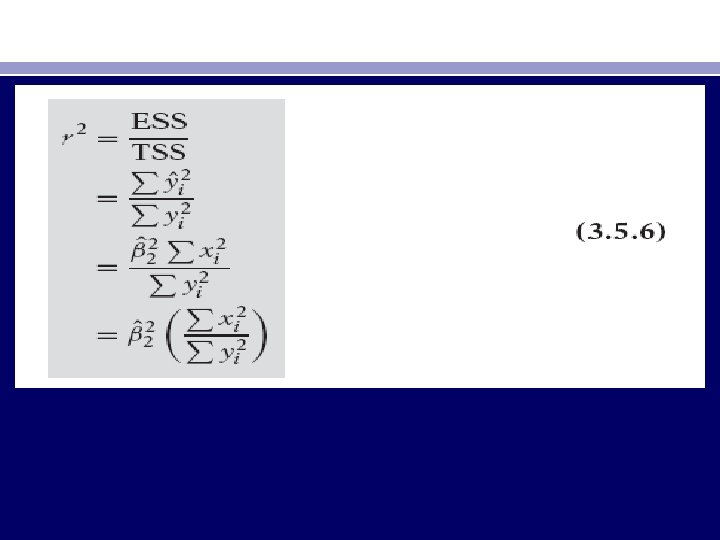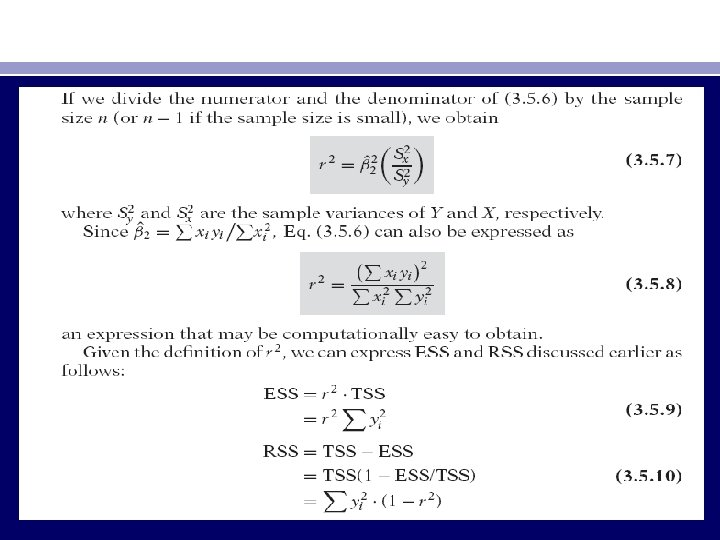• • Some of the properties of r are as follows (see Figure 3. 11): 1. It can be positive or negative, 2. It lies between the limits of − 1 and +1; that is, − 1 ≤ r ≤ 1. 3. It is symmetrical in nature; that is, the coefficient of correlation between X and Y(r. XY) is the same as that between Y and X(r. YX). 4. It is independent of the origin and scale; that is, if we define X*i = a. Xi + C and Y*i = b. Yi + d, where a > 0, b > 0, and c and d are constants, then r between X* and Y* is the same as that between the original variables X and Y. 5. If X and Y are statistically independent, the correlation coefficient between them is zero; but if r = 0, it does not mean that two variables are independent. 6. It is a measure of linear association or linear dependence only; it has no meaning for describing nonlinear relations. 7. Although it is a measure of linear association between two variables, it does not necessarily imply any cause-and-effect relationship.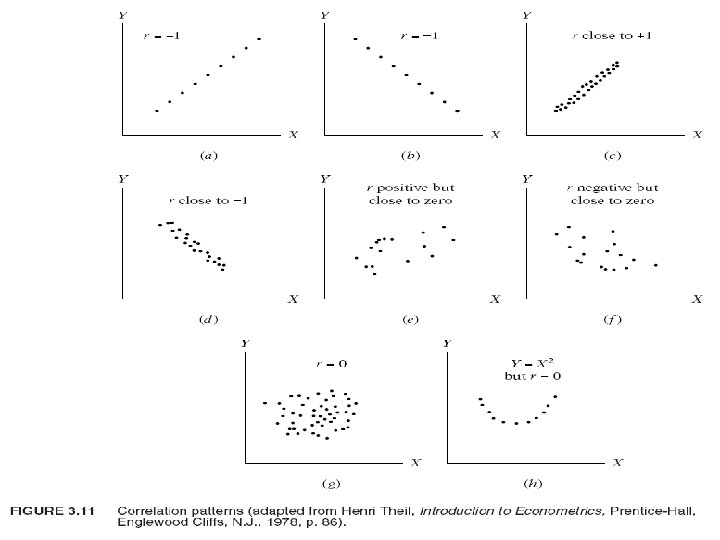• In the regression context, r 2 is a more meaningful measure than r, for the former tells us the proportion of variation in the dependent variable explained by the explanatory variable(s) and therefore provides an overall measure of the extent to which the variation in one variable determines the variation in the other. The latter does not have such value. Moreover, as we shall see, the interpretation of r (= R) in a multiple regression model is of dubious value. In passing, note that the r 2 defined previously can also be computed as the squared coefficient of correlation between actual Yi and the estimated Yi , namely, Yˆi. That is, using (3. 5. 13), we can write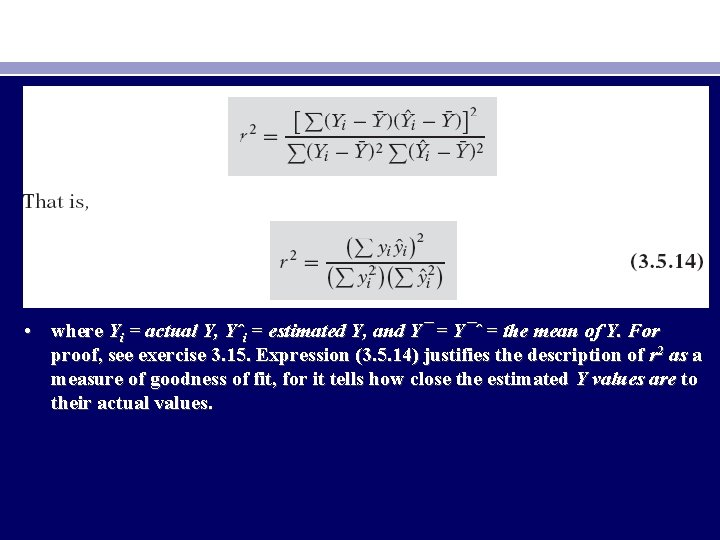• where Yi = actual Y, Yˆi = estimated Y, and Y¯ = Y¯ˆ = the mean of Y. For proof, see exercise 3. 15. Expression (3. 5. 14) justifies the description of r 2 as a measure of goodness of fit, for it tells how close the estimated Y values are to their actual values.A NUMERICAL EXAMPLE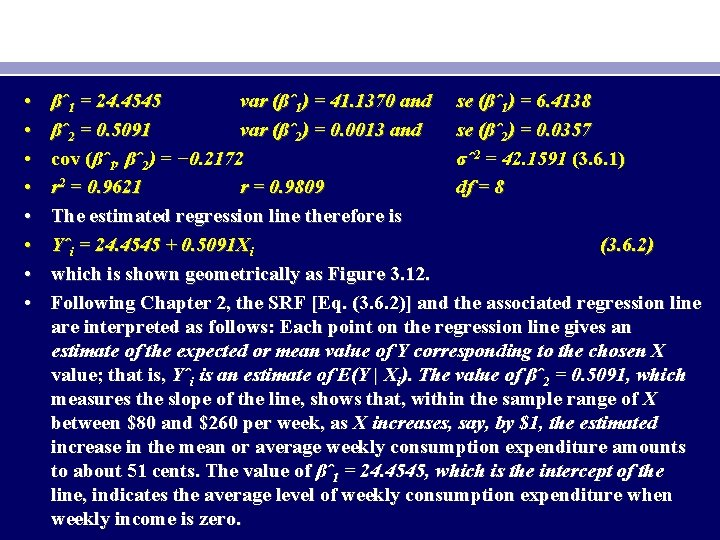• • βˆ1 = 24. 4545 var (βˆ1) = 41. 1370 and se (βˆ1) = 6. 4138 βˆ2 = 0. 5091 var (βˆ2) = 0. 0013 and se (βˆ2) = 0. 0357 cov (βˆ1, βˆ2) = − 0. 2172 σˆ2 = 42. 1591 (3. 6. 1) r 2 = 0. 9621 r = 0. 9809 df = 8 The estimated regression line therefore is Yˆi = 24. 4545 + 0. 5091 Xi (3. 6. 2) which is shown geometrically as Figure 3. 12. Following Chapter 2, the SRF [Eq. (3. 6. 2)] and the associated regression line are interpreted as follows: Each point on the regression line gives an estimate of the expected or mean value of Y corresponding to the chosen X value; that is, Yˆi is an estimate of E(Y | Xi). The value of βˆ2 = 0. 5091, which measures the slope of the line, shows that, within the sample range of X between \$80 and \$260 per week, as X increases, say, by \$1, the estimated increase in the mean or average weekly consumption expenditure amounts to about 51 cents. The value of βˆ1 = 24. 4545, which is the intercept of the line, indicates the average level of weekly consumption expenditure when weekly income is zero.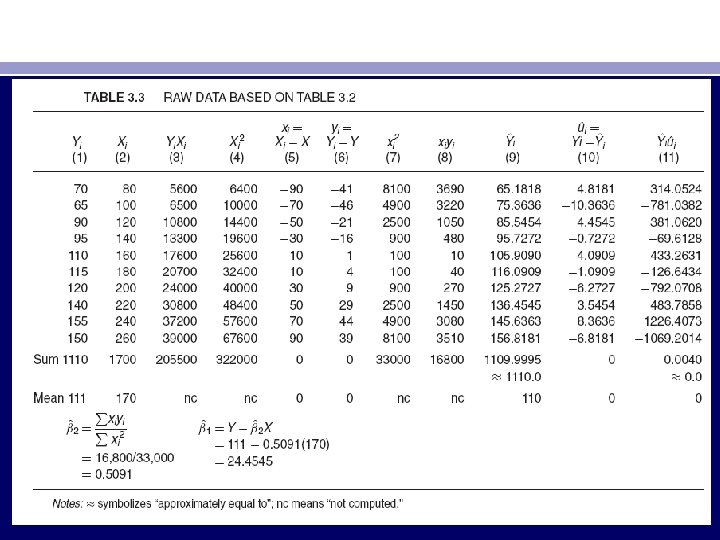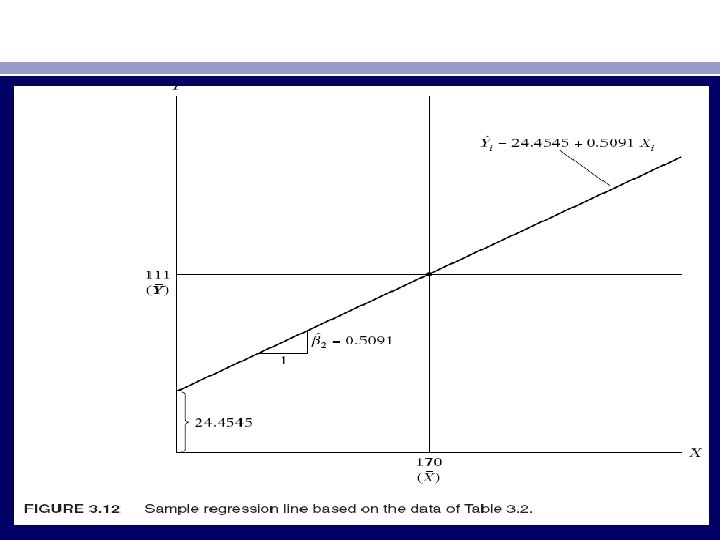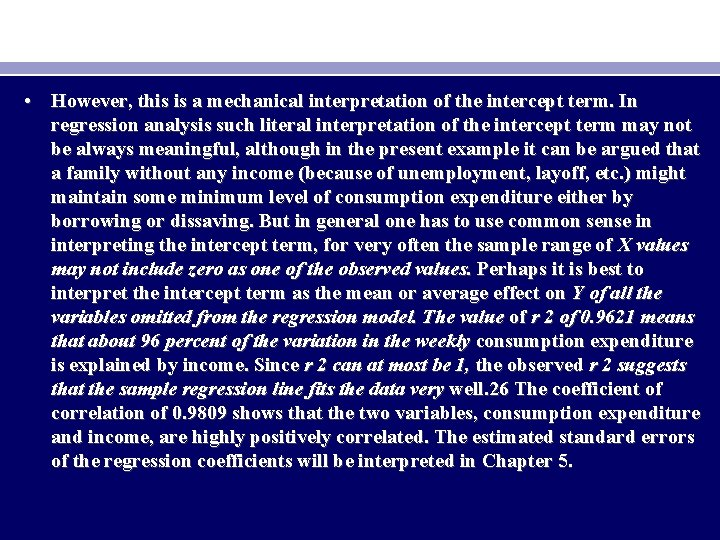• However, this is a mechanical interpretation of the intercept term. In regression analysis such literal interpretation of the intercept term may not be always meaningful, although in the present example it can be argued that a family without any income (because of unemployment, layoff, etc. ) might maintain some minimum level of consumption expenditure either by borrowing or dissaving. But in general one has to use common sense in interpreting the intercept term, for very often the sample range of X values may not include zero as one of the observed values. Perhaps it is best to interpret the intercept term as the mean or average effect on Y of all the variables omitted from the regression model. The value of r 2 of 0. 9621 means that about 96 percent of the variation in the weekly consumption expenditure is explained by income. Since r 2 can at most be 1, the observed r 2 suggests that the sample regression line fits the data very well. 26 The coefficient of correlation of 0. 9809 shows that the two variables, consumption expenditure and income, are highly positively correlated. The estimated standard errors of the regression coefficients will be interpreted in Chapter 5.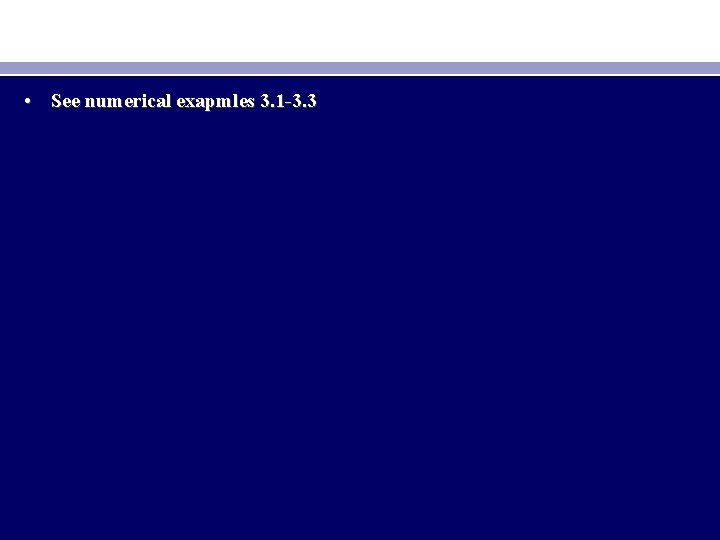• See numerical exapmles 3. 1 -3. 3